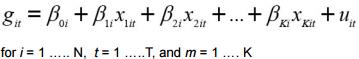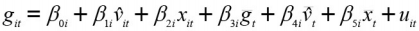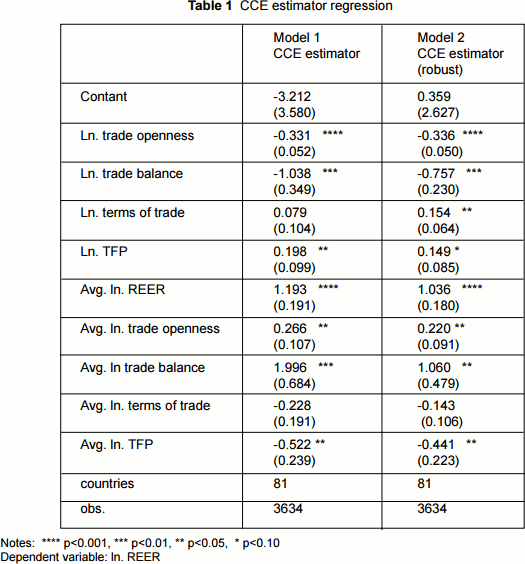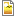# International trade and factor productivity as determinants of the real effective exchange rate

Authors: Ernesto R. Gantman and Marcelo P. Dabós

## Abstract

Using large panel data models that deal with the problems of potential endogeneity and cross-sectional dependence, while also allowing for cross-country heterogeneity in the parameters of interest, we demonstrate that trade openness produces a depreciation of the real effective exchange rate and total factor productivity leads to its appreciation.

## 1 Introduction

The study of the determinants of the real effective exchange rate (real effective exchange rate) has received much attention in the international economics literature, but the studies on this subject are not conclusive about the particular factors that affect the real effective exchange rate. This paper contributes to our knowledge about its determinants in a number of ways. First, since the empirical studies show that theoretical predictions hold for certain countries and not for others, our methodological approach does not restrict the slope coefficients of the independent variables to be the same for each country. Second, not only do we consider the issue of endogeneity, which arises as a result of simultaneous causation between the real effective exchange rate and trade openness, but we also deal with the problem of cross-sectional dependence, which to our knowledge has not been adequately addressed by the literature so far. We believe that cross-sectional correlation should be taken into account because movements in nominal exchange rates, and their potential concomitant effects on real exchanges rates,tend to affect countries not only individually, but also collectively — e.g., shocks to the US dollar. Third, our analysis improves over previous studies by using a new real effective exchange rate data set that covers a larger numbers of countries over an extended period of time, which provides enough empirical variability to observe changes of the real effective exchange rate under different economic conditions. Finally, the econometric technique employed is robust to the presence of potential relevant factors that may be unobserved.

## 2 The determinants of the real exchange rate

In our empirical analysis, we consider four determinants of the real effective exchange rate. The first one is trade openness.When the real effective exchange rate appreciates, domestic products become more expensive for the rest of the world and therefore their international demand decreases. In addition, foreign tradable products become cheaper than domestic ones, which increases imports unless the government raises their cost through tariffs. Thus,protectionism restricts imports and consolidates a situation of real effective exchange rate appreciation, and it has therefore been argued that trade liberalization leads to the opposite effect, a depreciation of the real effective exchange rate (Dornbusch1974). However, the empirical evidence about the relationship between trade openness and the real effective exchange rate has been mixed.

The terms of trade (a measure of the purchasing power of exports relative to imports) are also a factor that has been mentioned as a determinant of the real effective exchange rate. However, as Edwards and Wijnbergen (1987)argue, changes in terms of trade may generate a substitution and an income effect, which may operate in opposite directions. The empirical evidence is again mixed in this regard.

Another explanatory variable proposed in the literature is the difference in factor productivity. According to the Balassa-Samuelson effect, the real exchange rate will be overappreciated in richer countries (Balassa1964), and there has been some empirical evidence in favor of this effect.

It has also been suggested that capital inflows, which are reflected in the current account, tend to appreciate the real effective exchange rate. Unfortunately, we were not able to gather reliable comparative data on this variable.

We did include the trade balance, which for some authors can be considered a reasonable proxy for the current account (Doukas and Lifland, 1994). However, there is a potential problem of simultaneous causation in this regard, which we address in our econometric model, as an overappreciated real effective exchange rate tends to deteriorate the trade balance — it increases imports and decreases exports.

## 3 Data and methods

The dependent variable, real effective exchange rate (real effective exchange rate), is operationalized through the real effective exchange rate index elaborated by Darvas (2012), expressed in natural logarithms. Regarding the independent variables, trade openness is operationalized as the log of the sum of exports and imports asa percentage of the GDP, obtained from the World Development Indicators database of the World Bank(2015). For the terms-of-trade variable, we used the natural logarithm of an index that we computed dividing the price of exports index by the price of imports index from the Penn World Table 8.0 dataset (Feenstraet al., 2013). We calculated the trade balance indicator as the difference between exports and imports as a share of the GDP. We used the natural logarithm of this indicator, but we previously transformed the figures by adding 1 plus the minimum negative value in the dataset in order to avoid logs with null values.

Finally, we used the logarithm of the total factor productivity indicator from the Penn World Table. The sample only includes countries with a population greater than 1,000,000 and more than 30 consecutive yearly observations for all variables.

We use an econometric model of the form:The error term and the independent variables in (1) can be decomposed into random disturbances,time-variant and time-invariant unobserved factors, which can capture time-variant heterogeneity and cross-sectional dependence. Besides, an unobserved factor could influence both the error term and the observed regressors, thus inducing endogeneity (Eberhardt and Teal, 2011).

In equation (1), the dependent variable git is the log of the real effective exchange rate rate and k = 4, as we have fouri ndependent variables: trade openness, trade balance, terms of trade and factor productivity. The βki coefficients are country-specific slopes for each independent variable. To cope with the problem of cross sectional dependence, which otherwise could severely bias the regression coefficients, we used the Common Correlated Effects (CCE) Mean Group estimator introduced by Pesaran (2006), which utilizes an empirical augmentation of equation (1) that specifically addresses this issue as well as the potential presence of unobserved common factors in both the independent variables and the error term. In order to deal with these problems, the means of the dependent and all the independent variables are computed for each period and included as additional regressors in each individual country regression. Then, the coefficients obtained in the individual country regressions are averaged to give a consistent estimator of the observed variables. The CCE estimator is unaffected by unobserved common factors and robust to both endogeneity and the presence of non stationary common factors. Moreover, it does not require prior knowledge of the cointegrating properties of the observable or unobservable factors (Eberhardt and Teal2011).

However, an additional difficulty is the problem of potential simultaneity bias between the real effective exchange rate and(1) trade openness and (2) trade balance, which we have approached by instrumenting both independent variables with their first available lags in a two-step OLS framework, as proposed by Banerjee et al. (2010).

So, the final estimated model is the following:in which vit stands for the instrumented variables (trade openness and trade balance), which were instrumented with their first lags individually for each country, and xit represents the non-instrumented independent variables, while gt , vt , an xt are the means of the dependent variable, the instrumented variables and the non-instrumented independent variable, respectively. The final estimations were performed with the Stata xtmg routine (Eberhardt 2012).

## 4 Results and conclusion

Table 1 presents the results of the analysis. The CCE estimator (column 1) shows that trade openness has a negative and statistically significant effect on real effective exchange rate, suggesting that, as an economy increases its external trade as a share of the GDP, there is a real depreciation of its currency. The log of the trade balance also has a negative and a statistically significant effect (i.e., the lower the trade balance, the greater the real effective exchange rate over appreciation). The coefficient of the terms of trade is also aligned with theoretical expectations with a positive sign, but it lacks statistical significance. Finally, total factor productivity shows a positive and statistically significant relationship with the real effective exchange rate. The rest of the coefficients for the CCE model can be interpreted as nuisance parameters, which are simply incorporated to deal with the issue of cross sectional dependence. In column 2, we present a robust version of the CCE estimator (Bond et al.,2010), which uses weights for the values of the country regression parameters to diminish the influence of extreme values in the calculation of the average coefficients of the parameters. The results are very similar, except that the terms-of-trade variable is now statistically significant.As a test of robustness, we used an alternative estimator, the augmented mean group estimator of Eberhardt and Teal (2011), which also takes into account the issue of cross-sectional dependence, and the obtained results (not reported here) were qualitatively similar. In addition, we used as an alternative indicator the terms of trade from the World Bank WDI database (2016). With this indicator, the number of countries diminished to 49 and two variables, the trade balance and the terms of trade, were no longer statistically significant. Therefore, the only robust results correspond to trade openness and total factor productivity.

This study advances our empirical knowledge of the determinants of the real effective exchange rate by allowing heterogeneity in the parameters and recognizing the issue of cross-sectional dependence, which appears as a basic feature of an increasingly interconnected international economy. Our findings support the existence of a Balassa-Samuelson effect, as total factor productivity has a positive effect on the real effective exchange rate. In addition, there is also support for the hypothesis of a link between trade openness and the real effective exchange rate, supporting earlier thinking on this subject. However, since openness has been measured as the share of total international trade over GDP and not directly by looking at the specific trade policies adopted by the countries (e.g.,lowering tariffs, establishing trade agreements with other countries, abolishing quotas, subsidizing exports,etc.), the actual mechanisms by which trade openness affects the real effective exchange rate merit further research.

## References

Balassa, B. (1964). The Purchasing Power Parity Doctrine: A Reappraisal. Journal of Political Economy,
72(6), 584-596.
Banerjee, A., Eberhardt, M., & Reade, J. (2010). Panel Estimation for Worriers. WP514, University of
Oxford, Department of Economics.
Bond, S., Leblebicioglu, A., & Schiantarelli, F. (2010). Capital Accumulation and Growth: A New Look at
the Empirical Evidence. Journal of Applied Econometrics, 25(7),1073-1099.
Darvas, Z. (2012). Real effective exchange rates for 178 countries: A new database (Bruegel Working
Paper 2012/06). Brussels.
Dornbusch, R. (1974). Tariffs and Nontraded Goods. Journal of International Economics, 4(2), 177-185.
Doukas, J., & Lifland, S. (1994). Exchange Rates and the Role of the Trade Balance Account. Managerial
Finance, 20(5), 67-78.
Eberhardt, M. (2012). Estimating Panel Time-Series Models with Heterogeneous Slopes. The Stata
Journal, 12(1), 61-71.
Eberhardt, M., & Teal, F. (2011). Econometrics for Grumblers: A New Look at the Literature on CrossCountry
Growth Empirics. Journal of Economic Surveys, 25,109–155.
Edwards, S., & Van Wijinbergen, S. (1987). Tariffs, the Real Exchange Rate and the Terms of Trade: On
Two Popular Propositions in International Economics. Oxford Economics Papers, 39(3), 458-64.
Feenstra, R. C., Inklaar, R., & Timmer, M. (2013). The Next Generation of the Penn World Table.
www.ggdc.net/pwt. (accessed 10 april 2016)
Pesaran, M. H. (2006). Estimation and Inference in Large Heterogeneous Panels with a Multifactor Error
Structure. Econometrica, 74(4), 967–1012.
World Bank (2016). World Development Indicators.trade-1.pngtrade-2.pngtrade-3.png